Courses

# Viscosity and Laminar Flow; Poiseuille’s Law - Part 1 Physics Notes | EduRev

## Physics : Viscosity and Laminar Flow; Poiseuille’s Law - Part 1 Physics Notes | EduRev

The document Viscosity and Laminar Flow; Poiseuille’s Law - Part 1 Physics Notes | EduRev is a part of the Physics Course Basic Physics for IIT JAM.
All you need of Physics at this link: Physics

Laminar Flow and Viscosity
When you pour yourself a glass of juice, the liquid flows freely and quickly. But when you pour syrup on your pancakes, that liquid flows slowly and sticks to the pitcher. The difference is fluid friction, both within the fluid itself and between the fluid and its surroundings. We call this property of fluids viscosity. Juice has low viscosity, whereas syrup has high viscosity. In the previous sections we have considered ideal fluids with little or no viscosity. In this section, we will investigate what factors, including viscosity, affect the rate of fluid flow.
When you pour yourself a glass of juice, the liquid flows freely and quickly. But when you pour syrup on your pancakes, that liquid flows slowly and sticks to the pitcher. The difference is fluid friction, both within the fluid itself and between the fluid and its surroundings. We call this property of fluids viscosity. Juice has low viscosity, whereas syrup has high viscosity. In the previous sections we have considered ideal fluids with little or no viscosity. In this section, we will investigate what factors, including viscosity, affect the rate of fluid flow.
The precise definition of viscosity is based on laminar, or nonturbulent, flow. Before we can define viscosity, then, we need to define laminar flow and turbulent flow. Both types of flow. Laminar flow is characterized by the smooth flow of the fluid in layers that do not mix. Turbulent flow, or turbulence, is characterized by eddies and swirls that mix layers of fluid together.
Smoke rises smoothly for a while and then begins to form swirls and eddies. The smooth flow is called laminar flow, whereas the swirls and eddies typify turbulent flow. If you watch the smoke (being careful not to breathe on it), you will notice that it rises more rapidly when flowing smoothly than after it becomes turbulent, implying that turbulence poses more resistance to flow.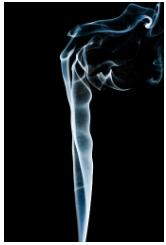Schematically how laminar and turbulent flow differ. Layers flow without mixing when flow is laminar. When there is turbulence, the layers mix, and there are significant velocities in directions other than the overall direction of flow. The lines that are shown in many illustrations are the paths followed by small volumes of fluids. These are called streamlines. Streamlines are smooth and continuous when flow is laminar, but break up and mix when flow is turbulent. Turbulence has two main causes. First, any obstruction or sharp corner, such as in a faucet, creates turbulence by imparting velocities perpendicular to the flow. Second, high speeds cause turbulence. The drag both between adjacent layers of fluid and between the fluid and its surroundings forms swirls and eddies, if the speed is great enough. We shall concentrate on laminar flow for the remainder of this section, leaving certain aspects of turbulence for later sections.
(a) Laminar flow occurs in layers without mixing. Notice that viscosity causes drag between layers as well as with the fixed surface.
(b) An obstruction in the vessel produces turbulence. Turbulent flow mixes the fluid. There is more interaction, greater heating, and more resistance than in laminar flow.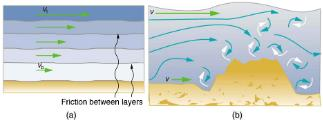Making Connections: Take-Home Experiment: Go Down to the River
Try dropping simultaneously two sticks into a flowing river, one near the edge of the river and one near the middle. Which one travels faster? Why?
How viscosity is measured for a fluid. Two parallel plates have the specific fluid between them. The bottom plate is held fixed, while the top plate is moved to the right, dragging fluid with it. The layer (or lamina) of fluid in contact with either plate does not move relative to the plate, and so the top layer moves at v while the bottom layer remains at rest. Each successive layer from the top down exerts a force on the one below it, trying to drag it along, producing a continuous variation in speed from v to 0 as shown. Care is taken to insure that the flow is laminar; that is, the layers do not mix. The motion in [link] is like a continuous shearing motion. Fluids have zero shear strength, but the rate at which they are sheared is related to the same geometrical factors A and L as is shear deformation for solids.
The graphic shows laminar flow of fluid between two plates of area A. The bottom plate is fixed. When the top plate is pushed to the right, it drags the fluid along with it.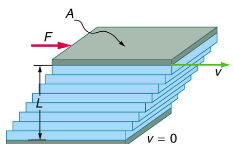A force F is required to keep the top plate moving at a constant velocity v, and experiments have shown that this force depends on four factors. First, F is directly proportional to v (until the speed is so high that turbulence occurs—then a much larger force is needed, and it has a more complicated dependence on v). Second, F is proportional to the area A of the plate. This relationship seems reasonable, since A is directly proportional to the amount of fluid being moved. Third, F is inversely proportional to the distance between the plates L. This relationship is also reasonable; L is like a lever arm, and the greater the lever arm, the less force that is needed. Fourth, F is directly proportional to the coefficient of viscosity, η. The greater the viscosity, the greater the force required. These dependencies are combined into the equation.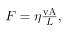which gives us a working definition of fluid viscosity η. Solving for η gives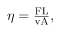which defines viscosity in terms of how it is measured. The SI unit of viscosity is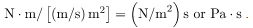The coefficients of viscosity for various fluids.
Viscosity varies from one fluid to another by several orders of magnitude. As you might expect, the viscosities of gases are much less than those of liquids, and these viscosities are often temperature dependent. The viscosity of blood can be reduced by aspirin consumption, allowing it to flow more easily around the body. (When used over the long term in low doses, aspirin can help prevent heart attacks, and reduce the risk of blood clotting.)
Laminar Flow Confined to Tubes—Poiseuille’s Law
What causes flow? The answer, not surprisingly, is pressure difference. In fact, there is a very simple relationship between horizontal flow and pressure. Flow rate Q is in the direction from high to low pressure. The greater the pressure differential between two points, the greater the flow rate. This relationship can be stated as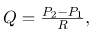where P1 and Pare the pressures at two points, such as at either end of a tube, and R is the resistance to flow. The resistance R includes everything, except pressure, that affects flow rate. For example, R is greater for a long tube than for a short one. The greater the viscosity of a fluid, the greater the value of R . Turbulence greatly increases R, whereas increasing the diameter of a tube decreases R.
If viscosity is zero, the fluid is frictionless and the resistance to flow is also zero. Comparing frictionless flow in a tube to viscous flow, we see that for a viscous fluid, speed is greatest at midstream because of drag at the boundaries. We can see the effect of viscosity in a Bunsen burner flame, even though the viscosity of natural gas is small.
The resistance R to laminar flow of an incompressible fluid having viscosity η through a horizontal tube of uniform radius r and length l, such as the one, is given by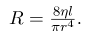This equation is called Poiseuille’s law for resistance after the French scientist J. L. Poiseuille (1799–1869), who derived it in an attempt to understand the flow of blood, an often turbulent fluid.
(a) If fluid flow in a tube has negligible resistance, the speed is the same all across the tube.
(b) When a viscous fluid flows through a tube, its speed at the walls is zero, increasing steadily to its maximum at the center of the tube.
(c) The shape of the Bunsen burner flame is due to the velocity profile across the tube.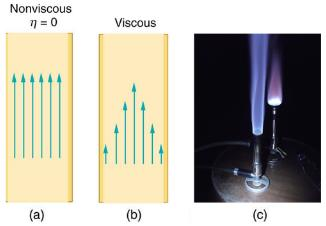Let us examine Poiseuille’s expression for R to see if it makes good intuitive sense. We see that resistance is directly proportional to both fluid viscosity η and the length l of a tube. After all, both of these directly affect the amount of friction encountered—the greater either is, the greater the resistance and the smaller the flow. The radius r of a tube affects the resistance, which again makes sense, because the greater the radius, the greater the flow (all other factors remaining the same). But it is surprising that r is raised to the fourth power in Poiseuille’s law. This exponent means that any change in the radius of a tube has a very large effect on resistance. For example, doubling the radius of a tube decreases resistance by a factor of 24 = 16.
Taken together,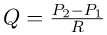and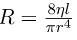give the following expression for flow rate: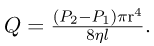This equation describes laminar flow through a tube. It is sometimes called Poiseuille’s law for laminar flow, or simply Poiseuille’s law.
Using Flow Rate: Plaque Deposits Reduce Blood Flow
Suppose the flow rate of blood in a coronary artery has been reduced to half its normal value by plaque deposits. By what factor has the radius of the artery been reduced, assuming no turbulence occurs?
Strategy
Assuming laminar flow, Poiseuille’s law states that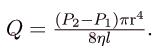We need to compare the artery radius before and after the flow rate reduction.
Solution
With a constant pressure difference assumed and the same length and viscosity, along the artery we have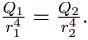So, given that Q2 = 0.5Q, we find that r2=0.5r14.
Therefore, r= (0.5)0.25r1=0.841r1 , a decrease in the artery radius of 16%.
Discussion
This decrease in radius is surprisingly small for this situation. To restore the blood flow in spite of this buildup would require an increase in the pressure difference (P2-P1) of a factor of two, with subsequent strain on the heart.
Coefficients of Viscosity of Various Fluids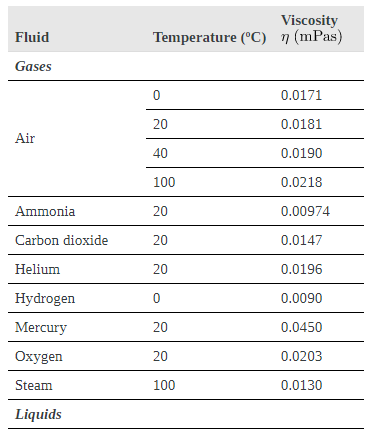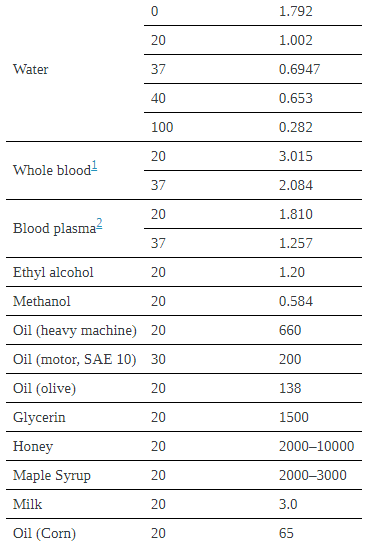The circulatory system provides many examples of Poiseuille’s law in action—with blood flow regulated by changes in vessel size and blood pressure. Blood vessels are not rigid but elastic. Adjustments to blood flow are primarily made by varying the size of the vessels, since the resistance is so sensitive to the radius. During vigorous exercise, blood vessels are selectively dilated to important muscles and organs and blood pressure increases. This creates both greater overall blood flow and increased flow to specific areas. Conversely, decreases in vessel radii, perhaps from plaques in the arteries, can greatly reduce blood flow. If a vessel’s radius is reduced by only 5% (to 0.95 of its original value), the flow rate is reduced to about (0.95)4 = 0.81 of its original value. A 19% decrease in flow is caused by a 5% decrease in radius. The body may compensate by increasing blood pressure by 19%, but this presents hazards to the heart and any vessel that has weakened walls. Another example comes from automobile engine oil. If you have a car with an oil pressure gauge, you may notice that oil pressure is high when the engine is cold. Motor oil has greater viscosity when cold than when warm, and so pressure must be greater to pump the same amount of cold oil.
Poiseuille’s law applies to laminar flow of an incompressible fluid of viscosity η through a tube of length l and radius r. The direction of flow is from greater to lower pressure. Flow rate Q is directly proportional to the pressure difference P2-P1, and inversely proportional to the length l of the tube and viscosity η of the fluid. Flow rate increases with r4, the fourth power of the radius.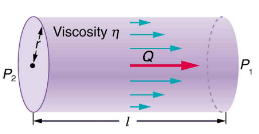What Pressure Produces This Flow Rate?
An intravenous (IV) system is supplying saline solution to a patient at the rate of 0.120 cm3/s through a needle of radius 0.150 mm and length 2.50 cm. What pressure is needed at the entrance of the needle to cause this flow, assuming the viscosity of the saline solution to be the same as that of water? The gauge pressure of the blood in the patient’s vein is 8.00 mm Hg. (Assume that the temperature i.)

Strategy
Assuming laminar flow, Poiseuille’s law applies. This is given by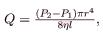where Pis the pressure at the entrance of the needle and P1 is the pressure in the vein. The only unknown is P2.
Solution
Solving for P2 yields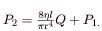P1 is given as 8.00 mm Hg, which converts to 1.066103N/m2. Substituting this and the other known values yields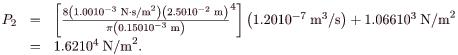Discussion
This pressure could be supplied by an IV bottle with the surface of the saline solution 1.61 m above the entrance to the needle (this is left for you to solve in this chapter’s Problems and Exercises), assuming that there is negligible pressure drop in the tubing leading to the needle.

Offer running on EduRev: Apply code STAYHOME200 to get INR 200 off on our premium plan EduRev Infinity!

## Basic Physics for IIT JAM

109 videos|157 docs|94 tests

,

,

,

,

,

,

,

,

,

,

,

,

,

,

,

,

,

,

,

,

,

;